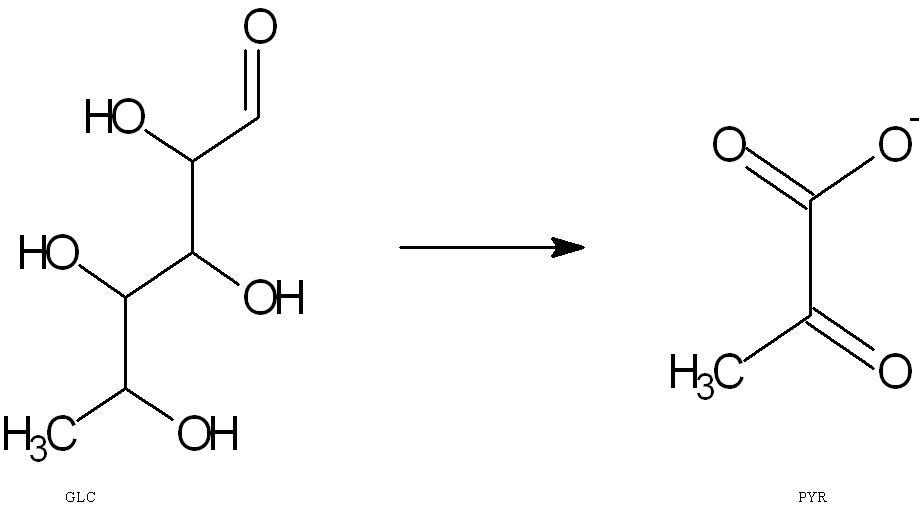# 3.2.5: Reaction Intermediates

$$\newcommand{\vecs}{\overset { \rightharpoonup} {\mathbf{#1}} }$$ $$\newcommand{\vecd}{\overset{-\!-\!\rightharpoonup}{\vphantom{a}\smash {#1}}}$$$$\newcommand{\id}{\mathrm{id}}$$ $$\newcommand{\Span}{\mathrm{span}}$$ $$\newcommand{\kernel}{\mathrm{null}\,}$$ $$\newcommand{\range}{\mathrm{range}\,}$$ $$\newcommand{\RealPart}{\mathrm{Re}}$$ $$\newcommand{\ImaginaryPart}{\mathrm{Im}}$$ $$\newcommand{\Argument}{\mathrm{Arg}}$$ $$\newcommand{\norm}{\| #1 \|}$$ $$\newcommand{\inner}{\langle #1, #2 \rangle}$$ $$\newcommand{\Span}{\mathrm{span}}$$ $$\newcommand{\id}{\mathrm{id}}$$ $$\newcommand{\Span}{\mathrm{span}}$$ $$\newcommand{\kernel}{\mathrm{null}\,}$$ $$\newcommand{\range}{\mathrm{range}\,}$$ $$\newcommand{\RealPart}{\mathrm{Re}}$$ $$\newcommand{\ImaginaryPart}{\mathrm{Im}}$$ $$\newcommand{\Argument}{\mathrm{Arg}}$$ $$\newcommand{\norm}{\| #1 \|}$$ $$\newcommand{\inner}{\langle #1, #2 \rangle}$$ $$\newcommand{\Span}{\mathrm{span}}$$$$\newcommand{\AA}{\unicode[.8,0]{x212B}}$$

A reaction intermediate is transient species within a multi-step reaction mechanism that is produced in the preceding step and consumed in a subsequent step to ultimately generate the final reaction product. Intermediate reactions are common in the biological world; a prime example can be seen in the metabolism of metabolites and nutrients.

## Introduction

The lifetime of an intermediate is usually short because it is usually consumed to make the next product of the reaction sequence. In a biochemical pathway, the overall reaction may seem to form only but product but may require multiple smaller steps to achieve that goal. It must be kept “in mind that an intermediate is always formed in an early elementary step and consumed in a later elementary step” (1).

##### Example $$\PageIndex{1}$$:

The general setup of a multi-step equation is:

$CH_4 + Cl_2 \rightarrow X \;\;\; \text{Step 1} \nonumber$

$X \rightarrow CH_3Cl + HCl \;\;\; \text{Step 2} \nonumber$

The first step yields a substance X, which is the intermediate of the chlorination reaction "formed on the pathway between reactants and products" (2).

##### Example $$\PageIndex{1}$$:

An example of a biological process is glycolysis. The overall balanced equation of glycolysis is

D- Glucose + NAD+ + 2 ADP + 2 Pi --> 2 Pyruvate + 2NADH + 2H+ +2ATP + 2H2OGLC --> PYR

Glucose (GLC) is the beginning reactant and is converted through a series of steps to form the final product, pyruvate (PYR). However, the intermediate “appears in the mechanism of the reaction but is not in the overall balanced equation.” One example of an intermediate in a glycolysis step reaction is the conversion of:Glucose --> Glucose-6-phosphate

RED = intermediate

The first step of glycolysis yields glucose-6-phosphate from a glucose molecule. However, in the overall balanced reaction of glycolysis, there is no presence of glucose-6-phosphate written because it exists for a short time before it is consumed in the next reaction.

## ProblemsIdentify and circle the intermediates of the glycolysis pathway and explain why they are considered intermediates. NOTE: *- DHAP is isomerized into G-3-P therefore the downstream products should be doubled.

## References

1. Chang, Raymond. Physical Chemistry for the Biological Sciences. Sausalito, California: University Science Books, 2005.Page 325
2. Shore, Neil E., and K. Peter C. Vollhardt. Organic Chemistry. 5th ed. New York, New York: W. H. Freeman and Company, 2007. Page 5